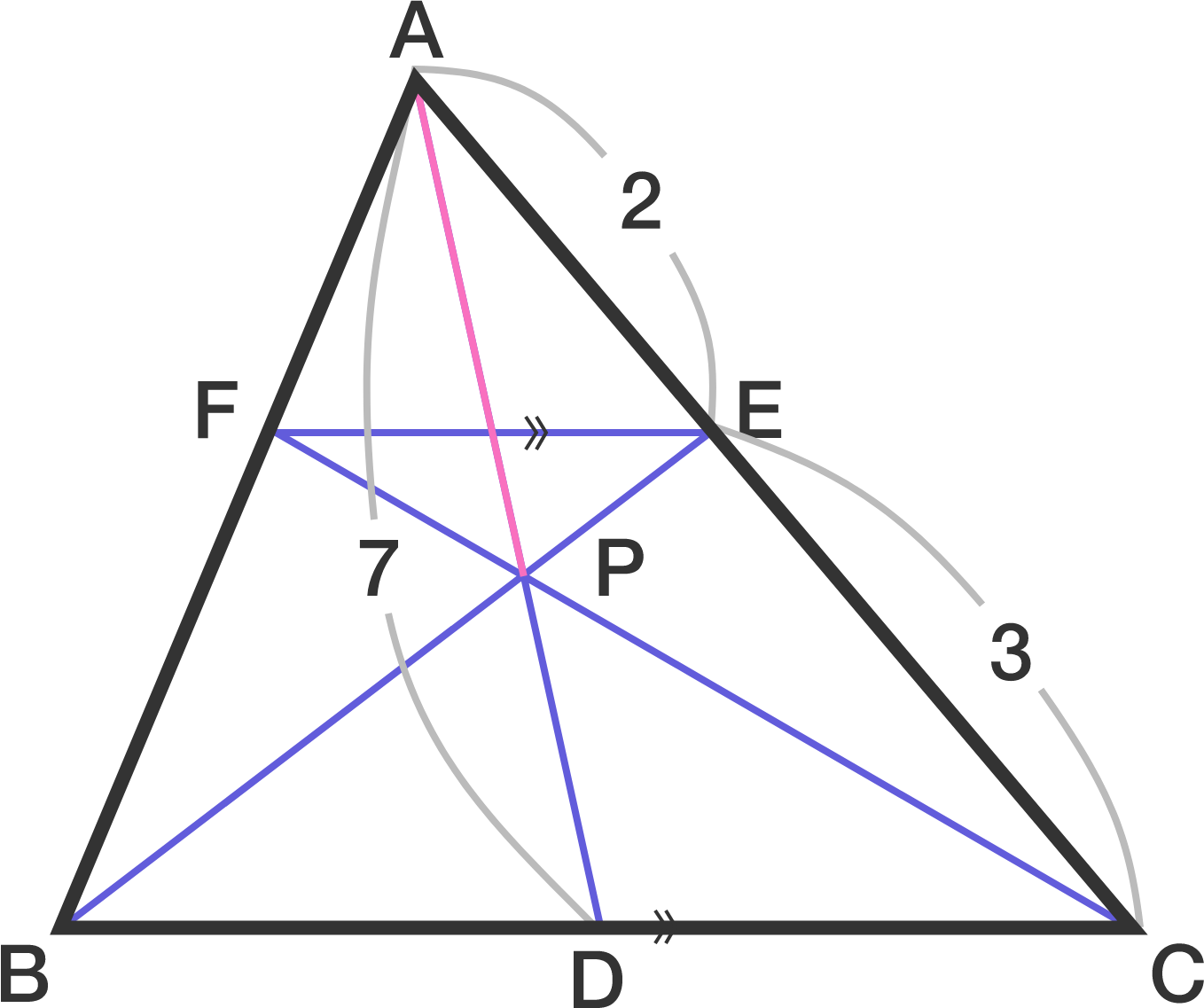# Ceva or Menelaus?

Geometry Level 2

In triangle $ABC,$ $E$ lies on $AC$ and $F$ lies on $AB$ such that $FE \parallel BC.$ $P$ is the intersection point of $BE$ and $CF,$ and extension of $AP$ meets $BC$ at point $D.$ Given that $AE=2, \quad CE=3, \quad AD=7,$ find length of $AP.$×